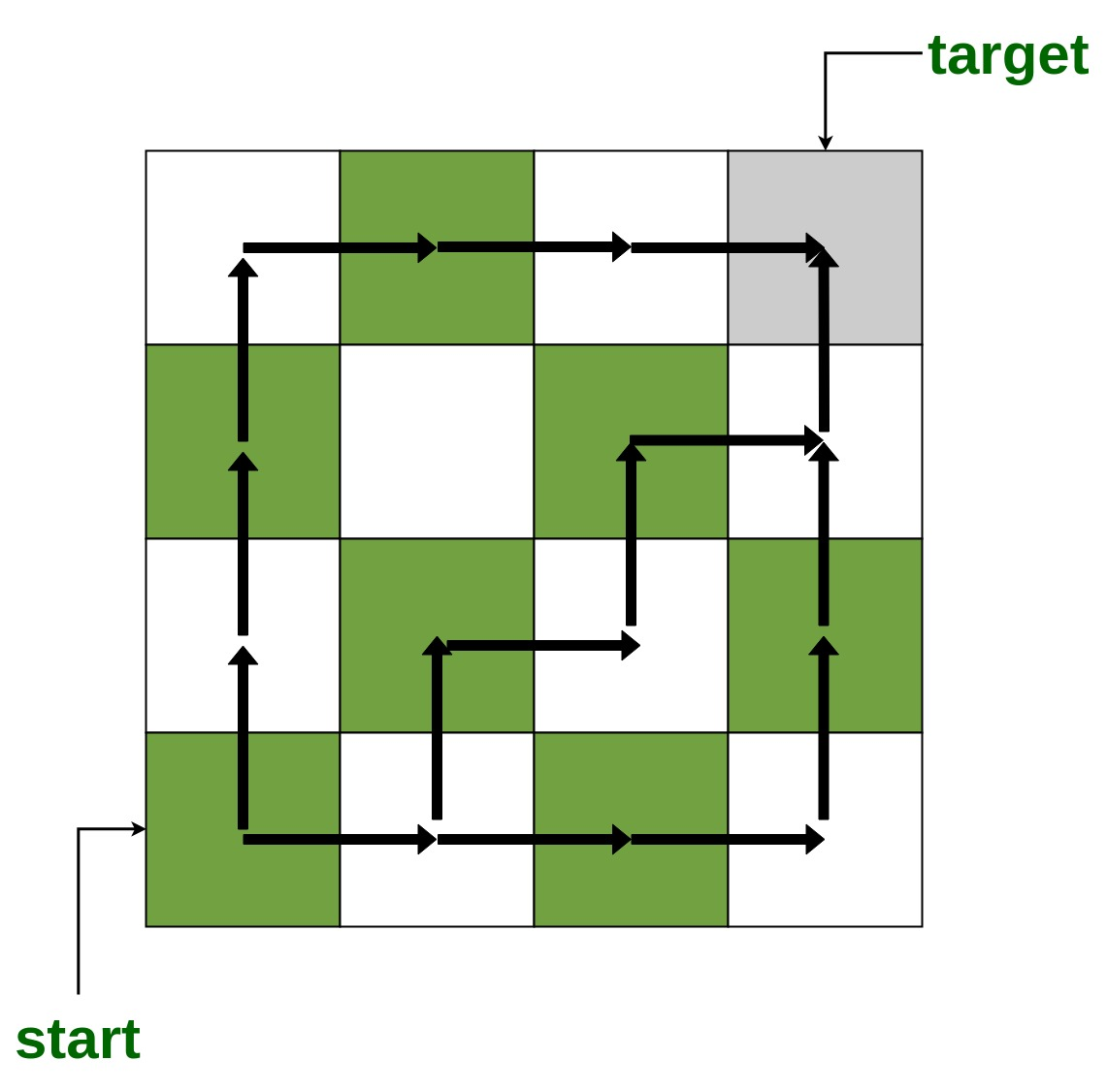Number of shortest paths to reach every cell from bottom-left cell in the grid

• Last Updated : 20 Sep, 2021

Given two number N and M. The task is to find the number of shortest paths to reach the cell(i, j) in the grid of size N × M when the moves started from the bottom-left corner
Note: cell(i, j) represents the ith row and jth column in the grid
Below image shows some of the shortest paths to reach cell(1, 4) in 4 × 4 gridExamples :

Input : N = 3, M = 4
Output : 1 3 6 10
1 2 3 4
1 1 1 1

Input : N = 5, M = 2
Output : 1 5
1 4
1 3
1 2
1 1

Approach : An efficient approach is to compute the grid starting from the bottom-left corner.

• The number of shortest paths to reach cell(n, i) is 1, where, 1 < = i < = M
• The number of shortest paths to reach cell(i, 1) is 1, where, 1 < = i < = N
• The number of shortest paths to reach cell(i, j) are the sum the number of shortest paths of cell(i-1, j) and (i, j+1), where, 1 < = j < = M and 1 < = i < = N

Below is the implementation of the above approach :

C++

 // CPP program to find number of shortest paths #include using namespace std;   // Function to find number of shortest paths void NumberOfShortestPaths(int n, int m) {     int a[n][m];       for (int i = 0; i < n; i++)         memset(a[i], 0, sizeof(a[i]));       // Compute the grid starting from     // the bottom-left corner     for (int i = n - 1; i >= 0; i--) {         for (int j = 0; j < m; j++) {             if (j == 0 or i == n - 1)                 a[i][j] = 1;             else                 a[i][j] = a[i][j - 1] + a[i + 1][j];         }     }       // Print the grid     for (int i = 0; i < n; i++) {         for (int j = 0; j < m; j++) {             cout << a[i][j] << " ";         }         cout << endl;     } }   // Driver code int main() {     int n = 5, m = 2;       // Function call     NumberOfShortestPaths(n, m);       return 0; }

Java

 // Java program to find number of shortest paths class GFG {   // Function to find number of shortest paths static void NumberOfShortestPaths(int n, int m) {     int [][]a = new int[n][m];       // Compute the grid starting from     // the bottom-left corner     for (int i = n - 1; i >= 0; i--)     {         for (int j = 0; j < m; j++)         {             if (j == 0 || i == n - 1)                 a[i][j] = 1;             else                 a[i][j] = a[i][j - 1] + a[i + 1][j];         }     }       // Print the grid     for (int i = 0; i < n; i++)     {         for (int j = 0; j < m; j++)         {             System.out.print(a[i][j] + " ");         }         System.out.println();     } }   // Driver code public static void main(String[] args) {     int n = 5, m = 2;       // Function call     NumberOfShortestPaths(n, m); } }   // This code is contributed by Princi Singh

Python3

 # Python 3 program to find # number of shortest paths   # Function to find number of shortest paths def NumberOfShortestPaths(n, m):     a = [[0 for i in range(m)]             for j in range(n)]       for i in range(n):         for j in range(m):             a[i][j] = 0       # Compute the grid starting from     # the bottom-left corner     i = n - 1     while(i >= 0):         for j in range(m):             if (j == 0 or i == n - 1):                 a[i][j] = 1             else:                 a[i][j] = a[i][j - 1] + \                           a[i + 1][j]           i -= 1       # Print the grid     for i in range(n):         for j in range(m):             print(a[i][j], end = " ")         print("\n", end = "")   # Driver code if __name__ == '__main__':     n = 5     m = 2       # Function call     NumberOfShortestPaths(n, m)       # This code is contributed by # Surendra_Gangwar

C#

 // C# program to find number of shortest paths using System;   class GFG {   // Function to find number of shortest paths static void NumberOfShortestPaths(int n, int m) {     int [,]a = new int[n, m];       // Compute the grid starting from     // the bottom-left corner     for (int i = n - 1; i >= 0; i--)     {         for (int j = 0; j < m; j++)         {             if (j == 0 || i == n - 1)                 a[i, j] = 1;             else                 a[i, j] = a[i, j - 1] + a[i + 1, j];         }     }       // Print the grid     for (int i = 0; i < n; i++)     {         for (int j = 0; j < m; j++)         {             Console.Write(a[i, j] + " ");         }         Console.Write("\n");     } }   // Driver code public static void Main(String[] args) {     int n = 5, m = 2;       // Function call     NumberOfShortestPaths(n, m); } }   // This code is contributed by PrinciRaj1992

Javascript



Output :

1 5
1 4
1 3
1 2
1 1

Time complexity: O(N × M)

My Personal Notes arrow_drop_up
Recommended Articles
Page :